# 题目描述（中等难度）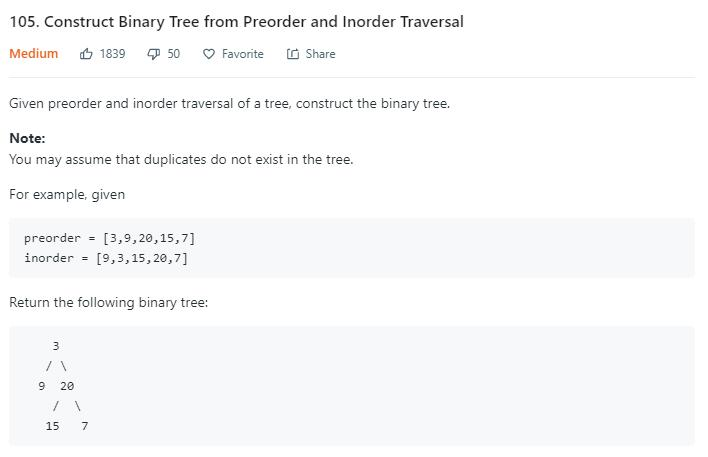# 解法一 递归

preorder = [3,9,20,15,7]
inorder = [9,3,15,20,7]

inorder 

inorder [15,20,7]

preorder
inorder 

preorder[20 15 7]
inorder [15,20,7]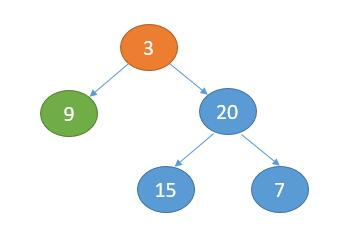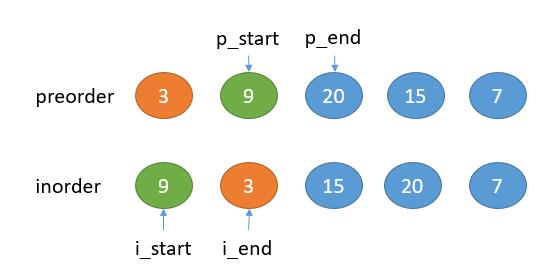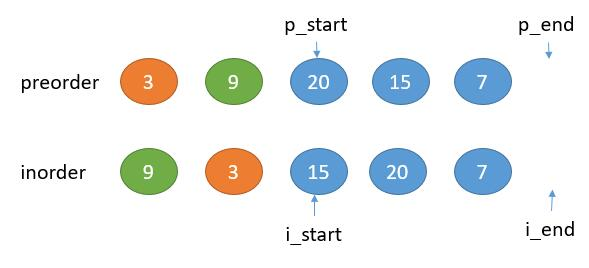public TreeNode buildTree(int[] preorder, int[] inorder) {
return buildTreeHelper(preorder, 0, preorder.length, inorder, 0, inorder.length);
}

private TreeNode buildTreeHelper(int[] preorder, int p_start, int p_end, int[] inorder, int i_start, int i_end) {
// preorder 为空，直接返回 null
if (p_start == p_end) {
return null;
}
int root_val = preorder[p_start];
TreeNode root = new TreeNode(root_val);
//在中序遍历中找到根节点的位置
int i_root_index = 0;
for (int i = i_start; i < i_end; i++) {
if (root_val == inorder[i]) {
i_root_index = i;
break;
}
}
int leftNum = i_root_index - i_start;
//递归的构造左子树
root.left = buildTreeHelper(preorder, p_start + 1, p_start + leftNum + 1, inorder, i_start, i_root_index);
//递归的构造右子树
root.right = buildTreeHelper(preorder, p_start + leftNum + 1, p_end, inorder, i_root_index + 1, i_end);
return root;
}


public TreeNode buildTree(int[] preorder, int[] inorder) {
HashMap<Integer, Integer> map = new HashMap<>();
for (int i = 0; i < inorder.length; i++) {
map.put(inorder[i], i);
}
return buildTreeHelper(preorder, 0, preorder.length, inorder, 0, inorder.length, map);
}

private TreeNode buildTreeHelper(int[] preorder, int p_start, int p_end, int[] inorder, int i_start, int i_end,
HashMap<Integer, Integer> map) {
if (p_start == p_end) {
return null;
}
int root_val = preorder[p_start];
TreeNode root = new TreeNode(root_val);
int i_root_index = map.get(root_val);
int leftNum = i_root_index - i_start;
root.left = buildTreeHelper(preorder, p_start + 1, p_start + leftNum + 1, inorder, i_start, i_root_index, map);
root.right = buildTreeHelper(preorder, p_start + leftNum + 1, p_end, inorder, i_root_index + 1, i_end, map);
return root;
}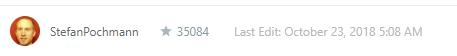pre变量保存当前要构造的树的根节点，从根节点开始递归的构造左子树和右子树，in变量指向当前根节点可用数字的开头，然后对于当前pre有一个停止点stop，从instop表示要构造的树当前的数字范围。

public TreeNode buildTree(int[] preorder, int[] inorder) {
return buildTreeHelper(preorder,  inorder, (long)Integer.MAX_VALUE + 1);
}
int pre = 0;
int in = 0;
private TreeNode buildTreeHelper(int[] preorder, int[] inorder, long stop) {
//到达末尾返回 null
if(pre == preorder.length){
return null;
}
//到达停止点返回 null
//当前停止点已经用了，in 后移
if (inorder[in] == stop) {
in++;
return null;
}
int root_val = preorder[pre++];
TreeNode root = new TreeNode(root_val);
//左子树的停止点是当前的根节点
root.left = buildTreeHelper(preorder,  inorder, root_val);
//右子树的停止点是当前树的停止点
root.right = buildTreeHelper(preorder, inorder, stop);
return root;
}


Consider the example again. Instead of finding the 1 in inorder, splitting the arrays into parts and recursing on them, just recurse on the full remaining arrays and stop when you come across the 1 in inorder. That's what my above solution does. Each recursive call gets told where to stop, and it tells its subcalls where to stop. It gives its own root value as stopper to its left subcall and its parents stopper as stopper to its right subcall.

      3
/   \
9     7
/ \
20  15

preorder = [ 3, 9, 20, 15, 7 ]
inorder = [ 20, 9, 15, 3, 7 ]
p 代表 pre，i 代表 in，s 代表 stop

s 初始化一个树中所有的数字都不会相等的数，所以代码中用了一个 long 来表示
3, 9, 20, 15, 7
^
p
20, 9, 15, 3, 7
^              ^
i              s

stop 值是当前根节点的值 3                只知道 stop 值是上次的 s

3, 9, 20, 15, 7                       3, 9, 20, 15, 7
^
p
20, 9, 15, 3, 7                       20, 9, 15, 3, 7
^          ^                                         ^
i          s                                         s

3, 9, 20, 15, 7
^
p
20, 9, 15, 3, 7
^
i
s



# 解法二 迭代 栈

      3
/   \
9     7
/ \
20  15


preorder = [3, 9, 20, 15, 7 ]


inorder = [ 20, 9, 15, 3, 7 ]


      3
/
9
/
20


1. 如果是 3 的右子树， 209 的右子树为空，那么中序遍历就是20 9 3 15

2. 如果是 9 的右子树，20 的右子树为空，那么中序遍历就是20 9 15

3. 如果是 20 的右子树，那么中序遍历就是20 15

     3
/
9
/ \
20  15


public TreeNode buildTree(int[] preorder, int[] inorder) {
if (preorder.length == 0) {
return null;
}
Stack<TreeNode> roots = new Stack<TreeNode>();
int pre = 0;
int in = 0;
//先序遍历第一个值作为根节点
TreeNode curRoot = new TreeNode(preorder[pre]);
TreeNode root = curRoot;
roots.push(curRoot);
pre++;
//遍历前序遍历的数组
while (pre < preorder.length) {
//出现了当前节点的值和中序遍历数组的值相等，寻找是谁的右子树
if (curRoot.val == inorder[in]) {
//每次进行出栈，实现倒着遍历
while (!roots.isEmpty() && roots.peek().val == inorder[in]) {
curRoot = roots.peek();
roots.pop();
in++;
}
//设为当前的右孩子
curRoot.right = new TreeNode(preorder[pre]);
//更新 curRoot
curRoot = curRoot.right;
roots.push(curRoot);
pre++;
} else {
//否则的话就一直作为左子树
curRoot.left = new TreeNode(preorder[pre]);
curRoot = curRoot.left;
roots.push(curRoot);
pre++;
}
}
return root;
}